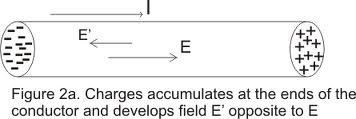# Current flow evenly in a conductor?

When current flows in a conductor, is it evenly spread out in the conductor? Not flowing(concentrated) in a certain part? And if we split the conductor into smaller pieces(visually not physically) the current divides out evenly so that when all those split pieces add up it equals the same as the whole piece?

Simon Bridge
Homework Helper
When current flows in a conductor, is it evenly spread out in the conductor?
No. Consider - the current consists of lots of same-sign charges on the move. Those charges will all repel each other won't they? So where will they tend to be concentrated?

•daviemmanuel
Well, I assumed they would be all spread out evenly, I can't think of a particle area they will be in a conductor(if not "all around") the edges then?

Simon Bridge
Homework Helper
I assumed they would be all spread out evenly,...
Yes but how?

When you draw electrostatic charges on a conductor, where do you normally draw them?

I went back and studied some old diagrams, of potential difference and the electric field produced on a conductor, I think... the electrostatics charge is spread out evenly on the edge of the conductor(bottom + and top - charges or vice versa), like this diagram:Simon Bridge
Homework Helper
Your question was about where the charges are while they are travelling as part of the current - that diagram does not tell you that.
But you have drawn the situation where there is a static charge distribution on a conductor and no applied electric field.
Where do you normally draw the charges?
Try sketching that case for a long cylinder.

The way I understand it, the uniformity with which the current distributes over the cross section of the conductor depends on the frequency of the signal.

For DC (direct current) or 0 Hz, the current carrying particles (electrons) tend to more evenly distribute over the entire cross section of the conductor.

When the signal starts being an oscillating, alternating one (AC), you introduce a changing magnetic field (induced electric field, induced currents, etc) ; when this happens, the electric current start by not using the center of the conductor and instead start crowding towards the outer layers of the conductor. With higher frequencies, the larger the "empty" inner area of the conductor and the thinner the outer layer effectively being used for carrying current. Research "skin effect".

So, for the case of alternating current, the answer is no...if you divide the conductor into wires, the current is not equally distributed...the wires from the center of the conductor could even be thrown away with no detectable effect other than material cost/weight savings.

•PhiowPhi, daviemmanuel and davenn
Simon Bridge
Homework Helper
•PhiowPhi
vanhees71
Gold Member
Well, it's a boundary-value problem of electrodynamics. For normal household DC, it's a good approximation to assume a homogeneous current density in the wire. Note that you can solve the infinite-length coax cable analytically, and that this is not done in most textbooks although you gain a lot of insights (also concerning energy flow). The best treatment of the problem I know is in

A. Sommerfeld, Lectures on Theoretical Physics, Vol. 3.

For AC you can treat the coax cable also analytically for the full Maxwell equations as a paradigmatic example for a wave-guide problem.

•PhiowPhi
We are not talking about excess free charge placed on a conductor (like a capacitor plate) which will move to the outside, the charge in a neutral (but conductive) wire is simply due to the charge carriers, and is completely neutralised over macroscopic scales by the positive ionic lattice. So you shouldn't see charge move to the outside for a simple wire due to any electrostatic effect.

However, the way those charges move is not necessarily balanced. If your wire has a kink, you will see a concentration of the current density at the inner part of the kink, like a car taking a 'racing line', because it is a path of less resistance to ground. As others have said, you also have a large concentration of current density to the outer surface with AC, which is an inductive effect.

But for a linear conductor excited by DC, the current density is indeed spread out homogeneously over any cross-section that is perpendicular to the direction of current.

Simon Bridge